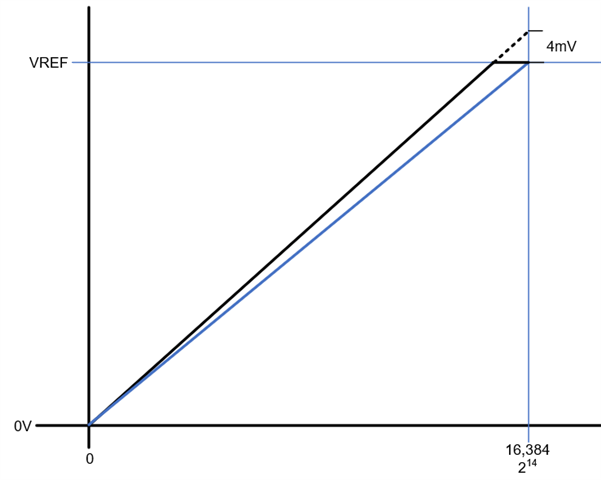If you have a related question, please click the "Ask a related question" button in the top right corner. The newly created question will be automatically linked to this question.

DAC8801: Calculation of the SIMPLE GAIN ERROR

Part Number: DAC8801

We would like to know the SIMPLE GAIN ERROR or DAC8801.
There is discription that "Full Scale Gain Error is 4mV (Maximum) in the datasheet.
1,Is this Full Scale Gain Error included another error such as Offcet error?
2,To calculate the max value of SIMPLE GAIN ERROR, how do I calculate it?

• Hello,

Full scale gain error is not included in offset error. Some of our datasheets give a spec for total unadjusted error (TUE) which is a combination of the DAC errors. You can learn more about TUE in this TI Precision Labs training video at the link here

I am unsure what you mean by simple gain error. The full scale gain error means that if the DAC voltage is 0V at zero code = 0V, then at full code the DAC will be a max of ±4mV off of the full scale voltage which is equal to the external reference voltage used. The error at any code can be calculated by finding the equation of the line based on the 4mV shift of the full scale voltage. in my example sketch below, the ideal DAC output function is given by the blue line, and the DAC output function with 4mV of gain error is given in black.Best,

Katlynne Jones